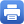Columbia Home
Mathematical Physics

The formulation of fundamental physical laws has always been tightly interwoven with the deepest mathematics available. This is even more evident today, with geometry providing the foundations of general relativity, gauge theories, string theory, and models of statistical physics. In turn, developments in these fields of physics have been instrumental in driving progress in various areas of mathematics, such as Riemann surface theory, quantum geometry, knot theory, mirror symmetry, representation theory, nonlinear partial differential equations and differential geometry. The cross-fertilization between mathematics and physics has perhaps never been richer.

A particularly active research area at Columbia is string theory, which is a candidate for a unified theory of all interactions, including a quantum theory of gravity. Of particular interest are the mathematical foundations of the theory, its eventual implications for phenomenology and cosmology, the geometry behind its dualities, and its intimate links with algebraic geometry, the theory of Riemann surfaces, and modular forms. Other active areas include supersymmetric gauge theories, integrable models, and the theory of solitons; and aspects of the theory of general relativity, particularly the study of Einstein’s equations and the mathematical theory of black holes.

The senior faculty group in mathematical physics consists of Brian Greene (string theory and cosmology), Duong H. Phong (string theory and differential geometry), Melissa Liu (symplectic geometry and general relativity), and Mu-Tao Wang (differential geometry and general relativity). The group interacts closely with the groups active in the topology and algebraic geometry of string theory and conformal field theory, consisting of Robert Friedman and Michael Thaddeus, and those interested in representation theory and topological quantum field theories, including Mikhail Khovanov, Andrei Okounkov, and Peter Woit. Other participating faculty include those from ISCAP, the Institute for Strings, Cosmology, and Astroparticle Physics.Print this page